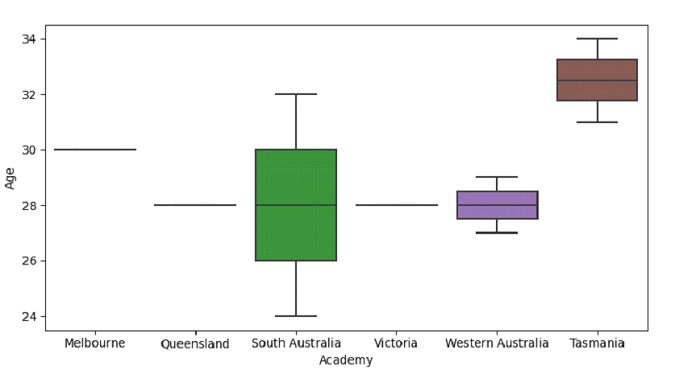# Python Pandas - Draw a vertical boxplot grouped by a categorical variable with Seaborn

Box Plot in Seaborn is used to draw a box plot to show distributions with respect to categories. To create a vertical Box Plot, use the seaborn.boxplot().

Let’s say the following is our dataset in the form of a CSV file − Cricketers.csv

At first, import the required libraries −

import seaborn as sb
import pandas as pd
import matplotlib.pyplot as plt

Load data from a CSV file into a Pandas DataFrame −

dataFrame = pd.read_csv("C:\Users\amit_\Desktop\Cricketers.csv")


Plotting a vertical box plot with Academy and Age −

sb.boxplot( x = 'Academy',y = 'Age', data = dataFrame )

## Example

Following is the complete code −

import seaborn as sb
import pandas as pd
import matplotlib.pyplot as plt

# Load data from a CSV file into a Pandas DataFrame:

# plotting a vertical box plot with Academy and Age
sb.boxplot( x = 'Academy',y = 'Age', data = dataFrame )

# display
plt.show()

## Output

This will produce the following output −Updated on: 01-Oct-2021

1K+ Views